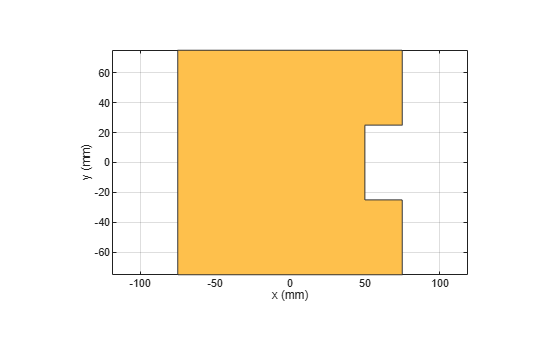subtract

Boolean subtraction operation on two shapes

Description

example

c = subtract(shape1,shape2) subtracts shape1 and shape2 using the subtract operation. You can also use the - to subtract the two shapes.

Examples

collapse all

Create a rectangle with a length of 0.15 m, and a width of 0.15 m.

r  = antenna.Rectangle('Length',0.15,'Width',0.15);

Create a second rectangle with a length of 0.05 m, and a width of 0.05 m. Set the center of the second rectangle at half the length of the first rectangle r.

n = antenna.Rectangle('Center',[0.075,0],'Length',0.05,'Width',0.05);

Create and view a notched rectangle by subtracting n from r.

rn  = r-n;
show(rn)Calculate the area of the notched rectangle.

area(rn)
ans = 0.0212

Input Arguments

collapse all

Shapes created using custom elements and shape objects of Antenna Toolbox™, specified as an object.

Example: c = subtract(rectangle1, rectangle2) where rectangle1 and rectangle2 are shapes created using antenna.Rectangle object.